Exotic looped trajectories of photons in three-slit interference

Abstract

The validity of the superposition principle and of Born’s rule are well-accepted tenants of quantum mechanics. Surprisingly, it has been predicted that the intensity pattern formed in a three-slit experiment is seemingly in contradiction with the most conventional form of the superposition principle when exotic looped trajectories are taken into account. However, the probability of observing such paths is typically very small, thus rendering them extremely difficult to measure. Here we confirm the validity of Born’s rule and present the first experimental observation of exotic trajectories as additional paths for the light by directly measuring their contribution to the formation of optical interference fringes. We accomplish this by enhancing the electromagnetic near-fields in the vicinity of the slits through the excitation of surface plasmons. This process increases the probability of occurrence of these exotic trajectories, demonstrating that they are related to the near-field component of the photon’s wavefunction.

Introduction

The phenomenon of interference has been recognized as ‘the only mystery’ of quantum mechanics1. The enormous interest and history of this fundamental effect can be traced back to the two-slit experiment devised by Thomas Young in the early 19th century. Young’s experiment is conceptually the simplest method for demonstrating the superposition principle, as the appearance of interference fringes in the far-field is unexplainable unless it is understood that the particle seemingly travels through both slits simultaneously. Such an experiment, originally performed with light, has since been conducted on particles ranging from individual photons, neutrons and atoms, to large molecules consisting of dozens of atoms2. As the superposition principle lies at the core of quantum physics, many of its counterintuitive features such as entanglement, non-locality, wave-particle duality, and delayed-choice concepts can be demonstrated or tested using a two-slit system3,4,5,6,7,8,9,10.

The standard interpretation of the two-slit experiment is given by solving the wave equation for an initially prepared complex wavefunction,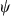. For example, if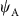represents the wavefunction at the detector for a photon emerging from slit A, and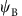is the wavefunction for a photon emerging from slit B, then the implementation of the superposition principle is to assume that the wavefunction is a superposition of the different paths given by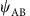=+. The probability of detection is given by Born’s rule as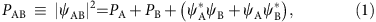where PA=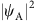and PB=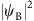. From this equation it is clear that the outcome of the two-slit experiment is given by the sum of outcomes from each slit alone, plus an additional interference term.

Due to the inherent structure of any wave theory, Born’s rule always bounds the complexity of any effect involving superpositions of an arbitrary number of wavefunctions to a sum of terms denoting the interference between pairs of wavefunctions11. For instance, in accordance with Born’s rule, the interference pattern obtained in a three-slit experiment can be described by the following probabilities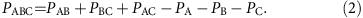Note that this expression does not include a probability term that involves three slits, but is entirely described by probabilities involving only one and two slits. Any possible contribution from higher-order interference terms (that is, a path involving the three slits) has been quantified by the so-called Sorkin parameter11,12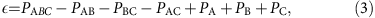which should be identically zero if only the direct paths through the three individual slits are considered. Sinha et al.12 showed that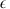can be evaluated experimentally by making a set of measurements for each term in equation (3).

Although it might appear that the measurement of a non-zeroimplies a clear violation of quantum mechanics12, De Raedt et al.13 demonstrated by numerically solving Maxwell’s equations that a non-zero value ofcan exist without such violation. Later it was found that this result is a consequence of the presence of exotic looped trajectories of light (for example, red curve in Fig. 1a) that arise in the Feynman path integral formulation with extremely low probability of occurrence14. This interpretation was subsequently shown to agree with the exact numerical solution of the wave equation15.Figure 1: Trajectories of light in a three-slit interferometer.

In this work, we demonstrate that looped trajectories of photons are physically due to the near-field component of the wavefunction, which leads to an interaction among the three slits. As such, it is possible to increase the probability of occurrence of these trajectories by controlling the strength and spatial distribution of the electromagnetic near-fields around the slits. By a proper control of the conditions in a three-slit experiment, we successfully demonstrate a dramatic increase of the probability of photons to follow looped trajectories, and present the first successful measurement of a non-zero value of.

Results

Origin of the looped trajectories of photons

Under the scalar wave approximation, the propagation of light is described by the Helmholtz equation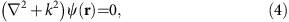subject to the boundary conditions specifying the physical setup. This equation can be solved by computing the propagation from any point r1 to any other point r2 via the Green’s function kernel, which according to Rayleigh-Sommerfeld theory is given by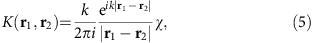where χ is an obliquity factor16. This equation satisfies equation (4) and the Fresnel-Huygens principle in the form of the following propagator relation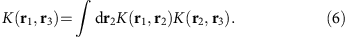If one repeatedly applies equation (6), the path-integral formulation of the propagation kernel is obtained in the form17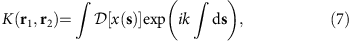where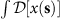is the functional integration over paths x(s). The boundary conditions can be included by restricting the possible paths x(s). If one is concerned only with diffraction from slits in a single plane, then equation (7) can be perturbatively expanded as14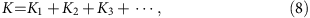where Kn represents the nth application of equation (6) and each integration is carried over the plane containing the slits (see Supplementary Note 1).

Solving the wave equation taking K=K1 is equivalent to considering only direct paths, such as the paths in Fig. 1b. These paths propagate from the source and through one of the slits to the detector. We call these wavefunctions,and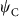. The higher-order terms in equation (8) are responsible for the looped trajectories of photons that propagate from the source to a slit, and to at least one other slit before propagating to the detector (see Fig. 1c). It follows that the wavefunction of a photon passing through the three slits is given by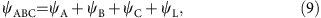where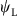represents the contribution of the looped trajectories to the wavefunction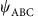. Note that in general, as defined by equation (3), is not zero because of the existence of these looped trajectories. Thus, the presence of looped paths leads to an apparent deviation of the superposition principle14.

Occurrence of looped trajectories of photons

The conclusion thatis not simply the superposition of the wavefunctions,andis a consequence of the actual boundary conditions in a three-slit structure. Changing the boundary conditions affects the near-field components around the slits, but it typically does not affect the far-field distribution because of the short range extension of the near fields18. As shown below, the looped trajectories of photons are physically due to the near-field components of the wavefunction. Therefore, by controlling the strengths and the spatial distributions of the near-fields around the slits, it is possible to drastically increase the probability of photons to undergo looped trajectories, thereby allowing a straightforward visualization of their effect in the far-field interference pattern. To demonstrate this phenomenon, a three-slit structure was designed such that it supports surface plasmons, which are strongly confined electromagnetic fields that can exist at the surface of metals19,20. The existence of these surface waves results in near fields that extend over the entire region covering the three slits6,21, thereby increasing the probability of looped trajectories.

As a concrete example, we consider the situations depicted in Fig. 1d,e. First, we assume a situation in which the incident optical field is a Gaussian beam polarized along the long axis of the slit (y polarization) and focused to a 400-nm spot size onto the left-most slit. For this polarization, surface plasmons are not appreciably excited and the resulting far-field distribution is the typical envelope, with no fringes, indicated by the dashed curve in Fig. 1e. This intensity distribution is described by the quantity. The presented results were obtained through a full-wave numerical analysis based on the finite-difference-time-domain (FDTD) method, on a structure with dimensions w=200 nm, p=4.6 μm, and t=110 nm and at a wavelength λ=810 nm (see Methods). The height of the slit, h, was assumed to be infinite. Interestingly, the situation is very different when the incident optical field is polarized along the x direction. The Poynting vector for this situation is shown in Fig. 1d. This result shows that the Poynting vector predominantly follows a looped trajectory such as that schematically represented by the solid path in Fig. 1c. The resulting far-field interference pattern, shown as the solid curve in Fig. 1e, is an example of the interference between a straight trajectory and a looped trajectory. Thus, it is clear that the naive formulation of the superposition principle does not provide an accurate description for the case where near fields are strongly excited.

Experimental implementation

First, we experimentally verify the role that looped trajectories have in the formation of interference fringes. For this purpose we exclusively illuminate one of the three slits. This experiment is carried out in the set-up shown in Fig. 2a. As shown in Fig. 1f, no interference fringes are formed when heralded single-photons illuminating the slit are y-polarized. Remarkably, when the illuminating photons are polarized along the x direction the visibility of the far-field pattern is dramatically increased, see Fig. 1g,h. This effect unveils the presence of looped trajectories. In our experiment, the contributions from looped trajectories are quantified through the Sorkin parameter by measuring the terms in equation (3). To this end, we measured the interference patterns resulting from the seven arrangements of slits depicted in Fig. 2b, thus the illumination field fills each arrangement of slits. In our experiment, we use heralded single-photons with wavelength of 810 nm produced via degenerate parametric down-conversion (see Methods). The single photons were weakly focused onto the sample, and the transmitted photons were collected and collimated by an infinity-corrected microscope objective (see Fig. 2c). The resulting interference pattern was magnified using a telescope and recorded using an intensified charge-coupled device (ICCD) camera, which was triggered by the detection event of the heralding photon22. The strength of the near fields in the vicinity of the slits was controlled by either exciting or not exciting surface plasmons on the structure through proper polarization selection of the incident photons.Figure 2: Experimental set-up utilized to measure exotic trajectories of light.

The scanning electron microscope images of the fabricated slits are shown in the first row of Fig. 3. The dimensions of the slits are the same as those used for the simulation in Fig. 1, with h=100 μm being much larger than the beam spot size (15 μm). The interference patterns obtained when the contribution from near-field effects is negligible (y polarization) are shown in the second row, while those obtained in the presence of a strong near fields in the vicinity of the slits (x polarization) are shown in the third row. These interference patterns are obtained by adding 60 background-subtracted frames, each of which is captured within a coincidence window of 7 nsec over an exposure time of 160 s (see insets in Fig. 3). Only the pattern for PAB is shown in Fig. 3 because PAB and PBC produce nearly identical patterns in the far field, a similar situation occurs for PA, PB and PC. The bottom panels show detail views of the interference patterns measured along an horizontal line.

Note that the intensities of the interference patterns (that is, the probability amplitudes) for the two polarizations scale differently for each arrangement of slits. This is shown by the ratios of the position-averaged probabilities, Px/Py, indicated at the bottom of Fig. 3. The significant changes in the probabilities obtained with x-polarized photons ultimately lead to a value ofthat significantly deviate from zero. This interesting effect is produced by constructive and destructive interference among looped trajectories, whose probability has been increased through the enhancement of the near fields (see Supplementary Note 2).

Discussion

We quantify the contribution from the looped trajectories through the normalized Sorkin parameter, defined as κ/Imax with Imax being the intensity at the central maximum of the three-slit interference pattern14. Both theoretical and experimental values of this parameter are shown in Fig. 4a. The theoretical values were obtained via FDTD simulations, while the experimental values were calculated from the results in Fig. 3. Clearly, we observe that when the near fields are not enhanced, the parameter κ is much smaller than the uncertainty associated with our measurements. However, when the near fields are enhanced, κ is dramatically increased due to the increased probability for the looped trajectories14, enabling the measurement of this parameter despite experimental uncertainties. Taking as a reference the central maximum of the κ profile, the experimental results indicate that the contribution of looped trajectories has been increased by almost two orders of magnitude.Figure 4: Quantifying the contribution of looped trajectories through the normalized Sorkin parameter, κ.

Finally, we show that it is possible to control the probability of photons undergoing looped trajectories by modifying the dimensions of the three slit structure or by changing the wavelength of the optical excitation. Figure 4b,c show theoretical predictions and experimental data at the central maximum for different slit parameters and wavelengths. These measurements were taken with classical light from a tunable diode laser. Figure 4b shows the normalized Sorkin parameter for a situation in which looped trajectories significantly contribute to the formation of interference fringes, whereas Fig. 4c shows the same parameter for a situation in which near-field effects, and consequently looped trajectories, are negligible. In general, we note that the theoretical and experimental results are in good agreement, with the observed discrepancies being attributed to experimental uncertainties due to imperfections in the fabricated sample and due to the limited dynamic range of the camera.

We have demonstrated that exotic looped paths occur as a physical consequence of the near-field component of the wave equation. As such, it is possible to control the probability of occurrence of such paths by controlling the strength and spatial distribution of the near-fields around the slits. By doing so, we have shown a drastic increase in the probability of photons to follow looped paths, leading to the first experimental observation of such exotic trajectories in the formation of interference fringes. Our work elucidates new properties of light that could be used to enrich protocols that rely on interference effects such as quantum random walks and quantum simulators3,23.

Methods

Sample design

Full-wave electromagnetic simulations were conducted using a Maxwell’s equation solver based on the finite difference time domain method (Lumerical FDTD). The dispersion of the materials composing the structure was taken into account by using their frequency-dependent permittivities. The permittivity of the gold film was obtained from ref. 24, the permittivity of the glass substrate (BK7) was taken from the manufacturer’s specifications, and the permittivity of the index matching fluid (Cargille oil Type B 16484) was obtained by extrapolation from the manufacturer’s specification.

Sample fabrication

The glass substrates are standard BK7 cover slips (SCHOTT multipurpose glass D 263 T eco Thin Glass) with a thickness of 170 μm, polished on both sides to optical quality. The substrate was ultrasonically cleaned for 2 h in 2% Hellmanex III alkaline concentrate solution and subsequently rinsed and sonicated in methyl ethyl ketone (MEK) denatured Ethanol and then in demineralized water. The gold films were evaporated directly onto the clean glass substrates with no additional adhesive layer using a Plassys MEB 550S e-beam evaporation system. The growth of the film thickness was monitored in-situ during the evaporation by a water cooled quartz micro-balance. The slit patterns were structured by Ga ion beam milling using a Tescan Lyra 3 GMU SEM/FIB system with a canion FIB column from Orsay Physics. Each slit pattern consisted of 100 μm long slits. While fabricating the different slit sets, proper focusing of the FIB was checked by small test millings and if needed the FIB settings were readjusted accordingly to provide a consistent and reproducible slit quality.

Experiment

We generate single photons by means of heralding a photon by a ‘partner’ photon detection from a photon pair source. The photon pairs were created in a spontaneous parametric down conversion process using a 2 mm-long type-I nonlinear crystal (periodically poled potassium titanyl phosphate. We pump the crystal with a blue 405 nm continuous-wave diode laser (200 mW), thereby creating degenerate photon pairs at 810 nm wavelength. Both photons are passed through a 3 nm band-pass filter, coupled into a single-mode fibre and split by a 50/50-fibre beams splitter, which led to a coincidence count rate of 40kHz. The heralding photon is detected with a single-photon avalanche photo diode. Its partner photon is delayed by a 22 m long fibre, send through the setup and imaged by an ICCD. The ICCD is operated in the external triggering mode (7 ns coincidence gate time), where the heralding detection signal is used as an external trigger, to ensure that only these single photon events are registered22. Note that due to the low coincidence count rate there is only one photon at a time in the experimental set-up. For experiments using a weak laser instead of heralded single photons, the ICCD was operated in the continuous mode, where the intensifier is permanently switched on.

For the case in which we used single photons, the idler photons are detected by an avalanche photodiode (APD) that heralds the detection of signal photons with an ICCD. We used either y- or x-polarized light which is selected by means of a polarizer and half-wave plate. The beam is weakly focused onto the arrangement of slits that is mounted on a motorized three-axis translation stage that can be displaced in small increments of 60 nm. An infinity-corrected oil-immersion microscope objective (NA=1.4, magnification of 60 × , working distance of 100 μm) was used to collect the light emerging from the slit patterns. The light collected by the objective was then magnified with a telescope and measured by an ICCD camera.

Data analysis

The background subtracted interference patterns were used to determine the magnitudes of κ shown in Fig. 4. In Fig. 4a, we show the values of κ, obtained in the single photon regime, for different positions of the detector. The deviation from the theory and the magnitude of the error bars are larger at the edges of the κ profile because the signal is low at the edges of the interference patters, which results in a noisier signal. On the other hand, the central maximum of the interference patterns permits a more reliable characterization of κ. The values of κ obtained for classical light as a fuction of the wavelength, shown in Fig. 4b,c, were calculated using central maximum of the interference patterns. For these cases, we used only the regions of central fringe having intensities within 70% of the peak value. The data was then used to obtain the mean value and standard deviations for κ.

Data availability

The data that support the findings of this study are available from the corresponding author upon reasonable request.

How to cite this article: Magaña-Loaiza, O. S. et al. Exotic looped trajectories of photons in three-slit interference. Nat. Commun. 7, 13987 doi: 10.1038/ncomms13987 (2016).

Publisher's note: Springer Nature remains neutral with regard to jurisdictional claims in published maps and institutional affiliations.

References

1. 1

Mandel, L. Quantum effects in one-photon and two-photon interference. Rev. Mod. Phys. 71, 6861–6897 (1999).

2. 2

Greenberger, D. M., Horne, M. A. & Zeilinger, A. Multiparticle interferometry and the superposition principle. Phys. Today 46, 22–34 (1993).

3. 3

Shadbolt, P., Mathews, J. C. F., Laing, A. & O’Brien, J. L. Testing foundations of quantum mechanics with photons. Nat. Phys. 10, 278–286 (2014).

4. 4

Scully, M. O., Englert, B. G. & Walther, H. Quantum optical tests of complementarity. Nature 351, 111–116 (1991).

5. 5

Kim, Y. H., Yu, R., Kulik, S. P., Shih, Y. & Scully, M. O. Delayed ‘choice’ quantum eraser. Phys. Rev. Lett. 84, 0031–9007 (1999).

6. 6

Schouten, H. F. et al. Plasmon-assisted two-slit transmission: young’s experiment revisited. Phys. Rev. Lett. 94, 053901 (2005).

7. 7

Kocsis, S. et al. Observing the average trajectories of single photons in a two-slit interferometer. Science 332, 1170–1173 (2011).

8. 8

Menzel, R., Puhlmann, D., Heuer, A. & Schleich, W. P. Wave-particle dualism and complementarity unraveled by a different mode. PNAS 109, 9314–9319 (2012).

9. 9

Bolduc, E., Leach, J., Miatto, F. M., Leuchs, G. & Boyd, R. W. A fair sampling perspective on an apparent violation of duality. PNAS 111, 9314–9319 (2014).

10. 10

Mahler, D. H. et al. Experimental nonlocal and surreal Bohmian trajectories. Sci. Adv. 2, e11501466 (2016).

11. 11

Sorkin, R. D. Quantum mechanics as quantum measure theory. Mod. Phys. Lett. A 09, 3119–3127 (1994).

12. 12

Sinha, U., Couteau, C., Jennewein, T., Laflamme, R. & Weihs, G. Ruling out multi-order interference in quantum mechanics. Science 329, 418–421 (2010).

13. 13

De Raedt, H. & Hess, K. Analysis of multipath interference in three-slit experiments. Phys. Rev. A 85, 012101 (2012).

14. 14

Sawant, R., Samuel, J., Sinha, A., Sinha, S. & Sinha, U. nonclassical paths in quantum interference experiments. Phys. Rev. Lett. 113, 120406 (2014).

15. 15

Sinha, A., Vijay, A. & Sinha, U. On the superposition principle in interference experiments. Sci. Rep. 5, 10304 (2015).

16. 16

Goodman, J. W. Introduction to Fourier Optics McGraw-Hill (1968).

17. 17

Feynman, R. P. & Hibbs, A. R. Quantum Mechanics and Path Integrals 3rd edn, (McGraw-Hill, 1965).

18. 18

Kowarz, M. W. Homogeneous and evanescent contributions in scalar near-field diffraction. Appl. Opt. 34, 3055–3063 (1995).

19. 19

Raether, H. Surface Plasmons on Smooth and Rough Surfaces and on Gratings Springer (1988).

20. 20

Barnes, W. L., Dereux, A. & Ebbesen, T. W. Surface plasmon subwavelength optics. Nature 424, 824–830 (2003).

21. 21

Gay, G. et al. The optical response of nanostructured surfaces and the composite diffracted evanescent wave model. Nat. Phys. 2, 262–267 (2006).

22. 22

Lapkiewicz, R., Ramelow, S., Fickler, R., Krenn, M. & Zeilinger, A. Real-time imaging of quantum entanglement. Sci. Rep. 3, 1914 (2013).

23. 23

Aspuru-Guzik, A. & Walther, P. Photonic quantum simulators. Nat. Phys. 8, 285–291 (2012).

24. 24

Johnson, P. & Christy, R. Optical constants of the noble metals. Phys. Rev. B 6, 4370–4379 (1972).

Acknowledgements

I.D.L., R.W.B. and R.F. gratefully acknowledge the support of the Canada Excellence Research Chairs Program. B.R. was supported by a MITRE Innovation Program Grant. R.F. also acknowledges the support of the Banting postdoctoral fellowship of the Natural Sciences and Engineering Research Council of Canada. U.M. acknowledges the support of DFG under GRK1896. The University of Rochester portion of the work was supported by KBN optics and internal university funds. O.S.M.-L. and R.W.B. thank P. Milonni, N. Bigelow, N. Vamivakas, Q. Lin and A. Liapis for helpful discussions and comments. We thank A. Gumann and T. Kreller for helping in the preparation of the gold coated glass substrates, and E. Karimi for providing the nonlinear crystal.

Author information

O.S.M.-L. conceived the idea with help from I.D.L. and R.W.B. The experiment was designed by I.D.L., O.S.M.-L. and R.W.B. The numerical analysis was carried out by I.D.L. The theoretical description of our work was developed by B.R. and M.M. The experiment was performed by O.S.M.-L., I.D.L, R.F. and A.S., the samples were fabricated by U.M., B.M., P.B., and G.L. The data was analysed by O.S.M.-L. and I.D.L. with help from B.R. and M.M. The project was supervised by G.L. and R.W.B. All authors contributed to the discussion of the results and to the writing of the manuscript.

Correspondence to Omar S Magaña-Loaiza or Israel De Leon.

Ethics declarations

Competing interests

The authors declare no competing financial interests.

Supplementary information

Supplementary Information

Supplementary Figures, Supplementary Notes and Supplementary References. (PDF 2576 kb)

Rights and permissions

Reprints and Permissions

• Fringe visibility of exotic trajectories for matter waves in a double-slit experiment

• C. H. S. Vieira
• , H. A. S. Costa
• , Gustavo de Souza
• , Marcos Sampaio
•  & I. G. da Paz

Modern Physics Letters A (2019)

• The double-slit interference of large molecule

• Xiang-Yao Wu
• , Ji-Ping Liu
• , Ji Ma
• , Xiao-Ru Zhang
• , Han Liu
• , Xiao-Jing Liu
• , Si-Qi Zhang
•  & Hong Li

Quantum Studies: Mathematics and Foundations (2019)

• The Double-Slit Electron Diffraction Experiment with Aharonov-Bohm Phase Effect Revisited and the Divergence in its Asymptotic Form

• D. Assafrão
• , C. C. Favarato
• , S. V. B. Gonçalves
•  & G. Simonelli

Brazilian Journal of Physics (2019)

• Multi-path interferometry using single photons

• J P Cotter
•  & R P Cameron

Journal of Physics Communications (2019)

• Measurement of the Photon-Plasmon Coupling Phase Shift

• Akbar Safari
• , Robert Fickler
• , Enno Giese
• , Omar S. Magaña-Loaiza
• , Robert W. Boyd
•  & Israel De Leon

Physical Review Letters (2019)